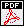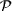## An Introduction in Discrete Time

Published in July 2002 by Walter de Gruyter , Berlin.

ix + 422 pages. Hardcover 24 x 17 cm.
€  54,- / sFr 86,- / for USA, Canada, Mexico US\$ 59,95

ISBN 3-11-017119-8

 For download:IntroductionTable of ContentsFlyer with order form

This book is an introduction to financial mathematics. It is intended for graduate students in mathematics and for researchers working in academia and industry.

The focus on stochastic models in discrete time has two immediate benefits. First, the probabilistic machinery is simpler, and one can discuss right away some of the key problems in the theory of pricing and hedging of financial derivatives. Second, the paradigm of a complete financial market, where all derivatives admit a perfect hedge, becomes the exception rather than the rule. Thus, the need to confront the problems arising in incomplete financial market models appears at a very early stage.

The first part of the book studies a simple one-period model which serves as a building stone for later developments. Topics include the characterization of arbitrage-free markets, the representation of preferences on asset profiles by expected utility theory and its robust extensions, monetary measures of risk, and an introduction to equilibrium analysis.

In the second part, the idea of dynamic hedging of contingent claims is developed in a multi-period framework. Such models are typically incomplete: They involve intrinsic risks which cannot be hedged away completely. Topics include martingale measures, pricing formulas for derivatives, American options, superhedging, and hedging strategies with minimal shortfall risk. Markets are modeled on general probability spaces. Thus, the text captures the interplay between probability theory and functional analysis which has been crucial for recent advances in mathematical finance.

Contents:

Part I: Mathematical finance in one period

1. Arbitrage theory
1.1 Assets, portfolios, and arbitrage opportunities
1.2 Absence of arbitrage and martingale measures
1.3 Derivative securities
1.4 Complete market models
1.5 Geometric characterization of arbitrage-free models
1.6 Contingent initial data

2. Preferences
2.1 Preference relations and their numerical representation
2.2 von Neumann-Morgenstern representation
2.3 Expected utility
2.4 Uniform preference
2.5 Robust preferences on asset profiles
2.6 Probability measures with given marginals

3. Optimality and equilibrium
3.1 Portfolio optimization and the absence of arbitrage
3.2 Exponential utility and relative entropy
3.3 Optimal contingent claims
3.4 Microeconomic equilibrium

4. Monetary measures of risk
4.1 Risk measures and their acceptance sets
4.2 Robust representation of convex risk measures
4.3 Convex risk measures on L
4.4 Value at Risk
4.5 Measures of risk in a financial market
4.6 Shortfall risk

Part II: Dynamic hedging

5. Dynamic arbitrage theory
5.1 The multi-period market model
5.2 Arbitrage opportunities and martingale measures
5.3 European contingent claims
5.4 Complete markets
5.5 The binomial model
5.6 Convergence to the Black-Scholes price

6. American contingent claims
6.1 Hedging strategies for the seller
6.2 Stopping strategies for the buyer
6.3 Arbitrage-free prices
6.4 Lower Snell envelopes

7. Superhedging
7.1-supermartingales and upper Snell envelopes
7.2 Uniform Doob decomposition
7.3 Superhedging of American and European claims
7.4 Superhedging with derivatives

8. Efficient hedging
8.1 Quantile hedging
8.2 Hedging with minimal shortfall risk

9. Hedging under constraints
9.1 Absence of arbitrage opportunities
9.2 Uniform Doob decomposition
9.3 Upper Snell envelopes
9.4 Superhedging and risk measures

10. Minimizing the hedging error
10.1 Local quadratic risk
10.2 Minimal martingale measures
10.3 Variance-optimal hedging

Appendix
A.1 Convexity
A.2 Absolutely continuous probability measures
A.3 The Neyman-Pearson lemma
A.4 The essential supremum of a family of random variables
A.5 Spaces of measures
A.6 Some functional analysis

Notes
References
List of symbols
Index

Send comment